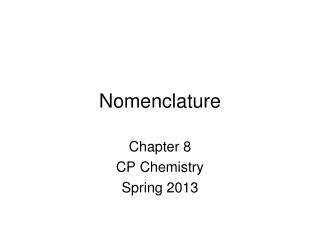DownloadDownload PresentationNomenclature

# Nomenclature

Télécharger la présentation## Nomenclature

- - - - - - - - - - - - - - - - - - - - - - - - - - - E N D - - - - - - - - - - - - - - - - - - - - - - - - - - -
##### Presentation Transcript

1. Nomenclature Chapter 8 CP Chemistry Spring 2013

2. Ionic Compounds • Atoms held together by ionic bonds. • What are ionic bonds? • Between metals and non-metals • Transfer of electrons between atoms. • Attraction between oppositely charged ions.

3. Ionic Compounds • Some examples are: • MgCl2 • MgCl2 • KBr • Li2O • These are all bonds between cations (positive ions) and anions (negative ions).

4. Naming Ionic Compounds • Binary Ionic Compounds • The metal will be your cation. Its name comes first. • The non-metal will be your anion. Its name comes second, but undergoes a slight change. • Change the ending to –ide. • Example: Chlorine  Chloride, Nitrogen  Nitride • Li2O Lithium + Ox ygen ide Metal Non-Metal

5. Practice • Name the following ionic compounds: • MgCl2 • Rb2S • BeF2 • Cs3N • KI • In ionic compounds, the subscripts do NOT affect the name of the compound. magnesium Chlor ine ide Rubidium Sulfide Beryllium Fluoride Cesium Nitride Potassium Iodide

6. Polyatomic Ions • Ions made of multiple atoms. • Name using an ion chart, but otherwise name the same as binary compounds. • Examples: • MgCO3 = Magnesium Carbonate • (NH4)2SO4 = Ammonium Sulfate

7. Writing Formulas from Names • The key to writing correct ionic formulas is to balance your positive and negative charges. We want a neutral molecule. • The “crossover method” is one strategy: The charge of the cation (metal ion) becomes the subscript of the anion. The charge of the anion (non-metal ion) becomes the subscript of the cation.

8. Writing Formulas From Names • Sodium Chloride • Sodium = Na+, Chlorine = Cl- Na1Cl1 NaCl

9. Writing Formulas from Names • Magnesium Fluoride • Magnesium = Mg2+, Fluorine = F- Mg1F2 MgF2 **Do not include the charge in your formula.

10. Why does this work? • Magnesium Fluoride Magnesium Fluorine -1 2+ -1 2 + -2 = 0

11. Ionic Naming using Transition Metals (and Tin and Lead) • The charges of transition metals can vary. SO use a ROMAN numeral to tell what the cation charge is. . . • You can use the formula to determine its charge in a specific molecule. • Writing Formulas From Names • You can write the formulas for ionic compounds with transition metals the same way as ionic compounds using alkali or alkaline earth metals. Mercury (I) Sulfide Mercury = Hg+1, Sulfur = S2- Hg2S

12. Example #1 • CuI2 • We know Iodine has a charge of -1, but we have to determine the charge of copper. Copper Iodine +? -1 So… the charge of Copper in this molecule must be +2. The name is written: Copper (II) Iodide -1 ____ + -2 = 0

13. Example #2 Chlorine Manganese • Mn2Cl4 +? -1 +? -1 We are trying to find the charge of one Manganese atom. The charge of one Manganese atom is +2, so the formula for Mn2Cl4 is: Manganese (II) Chloride -1 -1 2 x ? + -4 = 0

14. Practice • NiS2 • AgF • Sn3N4 • Lead (III) Phosphide • Titanium (IV) Chloride • Chromium (II) Iodide

15. Writing Formulas from Names • You can write the formulas for ionic compounds using transition metals the same as ionic compounds using alkali or alkaline earth metals. Mercury (I) Sulfide Mercury = Hg+, Sulfur = S2- Hg2S

16. Molecular Compounds • Held together by Covalent bonds. • Between two or more non-metals. • Sharing of electrons • Examples: • H2O • C2H4 • SO

17. Naming Molecular Compounds • Begin by writing the name as you would a binary ionic compound. • Add prefixes to each name which tell you how many of that atom are in the molecule. • If there is only one atom of the first element in the molecule you can leave out the “mono” prefix on the first element only. Prefix Definitions Mono = 1 Di = 2 Tri = 3 Tetra = 4 Penta = 5 Hexa = 6 Hepta = 7 Octa = 8 Nona = 9 Deca = 10

18. Practice • P2S3 • H2O • CO • N2O4

19. 2 non-metals (Carbon, Silicon, elements in groups 15-17, or Hydrogen) Metal + Non-metal or presence of polyatomic ion Includes Transition metal, Lead or Tin Includes Alkali or Alkaline Earth Metal Name using prefixes. Charges are not relevant. Must include charge of transition metal in parentheses. Name using binary ionic rules. No charge in parentheses needed.